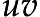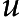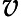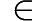Insertion sort "toposorts" when given a partial order

Posted on 2019-07-19 by Oleg Grenrus agda

I wonder: if we have a decidable partial ordering: like leq method in PartialOrd type class in lattices package.

Can we sort a list using leq so we get kind of a topological sorting?

Note, topological sorting is used for graphs: there we don’t have leq-like luxury. We’d first need to compute a transitive closure of a graph. On the other hand, given a list of "node", it may not include all nodes; and may include duplicates.

Insertion sort is a simple sorting algorithm, trivial to implement for lists (i.e. not in-place). I continue wondering: maybe it will produce topological ordering. Even insertion sort is simple, I still had an uneasy feeling: does it really work. Insertion sort terminates despite what function you pass as a comparator (even impure random one!), so what kind of output it produces when given lawful leq?

I must admit that exposure to Haskell and Agda made me suspect all pen and paper proofs, especially made by myself. So let’s certify insertion sort. The plan is to show how to certify insertion order first for total order and then for partial order.

#Certifying insertion sort

Module definitions and imports:

module Topo where

open import Data.List
open import Relation.Nullary
open import Relation.Binary.PropositionalEquality

Next, the definition of insert and sort. These are straight-forward and are familiar. Note that _≤?_ decides whether -relation holds. Returning Dec doesn’t forget what we compute (it returns the evidence), compared with simply returning Bool.

module Sort {A : Set} (_≤_ : A  A  Set)
(_≤?_ : (x y : A)  Dec (x ≤ y))
where
insert : A  List A  List A
insert x [] = x  []
insert x (y  ys) with x ≤? y
... | yes x≤y = x  y  ys
... | no ¬x≤y = y  insert x ys

sort : List A  List A
sort []       = []
sort (x  xs) = insert x (sort xs)

Twan van Laarhoven proved complete correctness of various sort algorithms (code gist). We’ll only do a sortedness of insertion sort.

Twan uses _≤?_ : (x y : A) (x ≤ y ⊎ y ≤ x) comparator ( is Either), that implies that ordering have to be total. Our ... Dec (x ≤ y) version is less powerful, therefore we’ll need to assume -flip.

Twan also defines insert to operate on Sorted xs, our proof is completely external.

There are few auxiliary lemmas, culminating with lemma that insert preserves sortedness, and the theorem that sort produces a sorted list.

One could also show that sort produces a permutation of input list, but that’s something I’m quite confident about already. Note: the proof of that fact won’t need any additional assumptions about .

By the way, these proofs show how dependently typed programming is full with various lists.1 Luckily (or not) Agda allows reuse of constructor names.

module Total (A : Set) (_≤_ : A  A  Set)
(_≤?_ : (x y : A)  Dec (x ≤ y))
(≤-trans :  {x y z}  x ≤ y  y ≤ z  x ≤ z)
(≤-flip  :  {x y}  ¬ (x ≤ y)  y ≤ x) -- precise enough!
where

infix 4 _≤*_

open Sort _≤_ _≤?_

data _≤*_ (x : A) : List A  Set where
[]  : x ≤* []
__ :  {y ys}  x ≤ y  x ≤* ys  x ≤* y  ys

data Sorted : List A  Set where
[] : Sorted []
__ :  {x xs}  x ≤* xs  Sorted xs  Sorted (x  xs)

*-trans :   x y ys  x ≤ y  y ≤* ys  x ≤* ys
*-trans x y []        x≤y []             = []
*-trans x y (y'  ys) x≤y (y≤y'  y'≤ys) =
-trans x≤y y≤y'  (≤*-trans x y ys x≤y y'≤ys)

lem-cons-all≤ :  x y ys  x ≤ y  y ≤* ys  x ≤* y  ys
lem-cons-all≤ x y ys x≤y y≤ys = x≤y *-trans x y ys x≤y y≤ys

lem-skip :  x y ys  y ≤ x  y ≤* ys  y ≤* insert x ys
lem-skip x y []        y≤x y≤ys        = y≤x  y≤ys
lem-skip x y (y'  ys) y≤x (y≤y'  y'≤ys ) with x ≤? y'
... | yes x≤y' = y≤x  y≤y'  y'≤ys
... | no ¬x≤y' = y≤y'  (lem-skip x y ys y≤x y'≤ys)

lem-insert-sorted :  x xs  Sorted xs  Sorted (insert x xs)
lem-insert-sorted x [] s = []  s
lem-insert-sorted x (y  ys) (y≤ys  sys) with x ≤? y
... | yes x≤y = lem-cons-all≤ x y ys x≤y y≤ys  y≤ys  sys
... | no ¬x≤y = lem-skip x y ys (≤-flip ¬x≤y) y≤ys  sorted-insert-x-ys
where sorted-insert-x-ys = lem-insert-sorted x ys sys

thm-sort-sorted :  xs  Sorted (sort xs)
thm-sort-sorted []       = []
thm-sort-sorted (x  xs) =
lem-insert-sorted x (sort xs) (thm-sort-sorted xs)

#... for only partial order

But what about partial order? Wikipedia says following about the topological sort:

In computer science, a topological sort or topological ordering of a directed graph is a linear ordering of its vertices such that for every directed edgefrom vertexto vertex,comes beforein the ordering.

We massage that into simple "there aren’t edges pointing backwards". So instead of saying: "for all sublists x ys, x is less-than-or-equal than any of yys" we say "for all subsets x ys, x is not greater-than any of yys".

After that, the proof structure is quite similar. I needed to use antisymmetry of , which shows that this Sorted predicate won’t hold for preorder. I’m not sure whether insertion order would work for preorder, I’m not sure it won’t work either.

module Partial (A : Set) (_≤_ : A  A  Set)
(_≤?_ : (x y : A)  Dec (x ≤ y))
(≤-trans :  {x y z}  x ≤ y  y ≤ z  x ≤ z)
(≤-antisym :  {x y}  x ≤ y  y ≤ x  x ≡ y)
where

open Sort _≤_ _≤?_

record _<_ (x y : A) : Set where
constructor le
field
is-le : x ≤ y
not-eq : ¬ (x ≡ y)

open _<_

<-trans₁ :  {x y z}  x < y  y ≤ z  x < z
<-trans₁ (le x≤y ¬x≡y) y≤z = le
(≤-trans x≤y y≤z)
(λ x≡z  ¬x≡y (≤-antisym x≤y (subst (λ i  _ ≤ i) (sym x≡z) y≤z)))

infix 4>*_

-- x ¬>* ys = x is not larger than any in y ∈ ys
data>*_ (x : A) : List A  Set where
[]  : x ¬>* []
__ :  {y ys}  ¬ y < x  x ¬>* ys  x ¬>* y  ys

data Sorted : List A  Set where
[] : Sorted []
__ :  {x xs}  x ¬>* xs  Sorted xs  Sorted (x  xs)

lem-trans-none> :   x y ys  x ≤ y  y ¬>* ys  x ¬>* ys
lem-trans-none> x y []       x≤y [] = []
lem-trans-none> x y (z  zs) x≤y (¬z<y  y≤zs) =
(λ z<y  ¬z<y (<-trans₁ z<y x≤y))  lem-trans-none> x y zs x≤y y≤zs

lem-flip :  {x y}  x ≤ y  ¬(y < x)
lem-flip {x} {y} x≤y with y ≤? x
... | yes y≤x = λ y<x  not-eq y<x (≤-antisym y≤x x≤y)
... | no ¬y≤x = λ y<x  ¬y≤x (is-le y<x)

lem-cons-none> :  x y ys  x ≤ y  y ¬>* ys  x ¬>* y  ys
lem-cons-none> x y ys x≤y y≤ys =
lem-flip x≤y  lem-trans-none> _ _ _ x≤y y≤ys

lem-skip :  x y ys  ¬ (x ≤ y)  y ¬>* ys  y ¬>* insert x ys
lem-skip x y []       ¬x≤y []   = (λ p  ¬x≤y (is-le p))  []
lem-skip x y (z  zs) ¬x≤y (¬z<y  y≤zs) with x ≤? z
... | yes x≤z = (λ x<y  ¬x≤y (is-le x<y))  ¬z<y  y≤zs
... | no ¬x≤z = ¬z<y  (lem-skip x y zs ¬x≤y y≤zs)

lem-insert-sorted :  x xs  Sorted xs  Sorted (insert x xs)
lem-insert-sorted x [] s = []  s
lem-insert-sorted x (y  ys) (y≤ys  sys) with x ≤? y
... | yes x≤y = lem-cons-none> x y ys x≤y y≤ys  y≤ys  sys
... | no ¬x≤y = lem-skip x y ys ¬x≤y y≤ys  sorted-insert-x-ys
where sorted-insert-x-ys = lem-insert-sorted x ys sys

thm-sort-sorted :  xs  Sorted (sort xs)
thm-sort-sorted []       = []
thm-sort-sorted (x  xs) =
lem-insert-sorted x (sort xs) (thm-sort-sorted xs)

Would merge sort work with partial order? I don’t know yet!

Let’s try in Haskell. You should try your hypothesis first, before trying to formally prove them. Proving false statements can take a lot of time!

After a little of imports, we’ll defined isSorted check, and try it on a N5 lattice. insertionSort works with leq, but a mergeSort only with <=.

import Algebra.PartialOrd
import Algebra.Lattice.N5
import Algebra.Lattice.Ordered
import Data.List (sortBy)
import Test.QuickCheck

lt :: PartialOrd a =>  a -> a -> Bool
lt x y = x /= y && leq x y

notGt :: PartialOrd a => a -> a -> Bool
notGt x y = not (lt y x)

-- | This checks that list is sorted in PartialOrd sense.
isSorted :: PartialOrd a => [a] -> Bool
isSorted []       = True
isSorted (x : ys) = isSorted ys && all (notGt x) ys

-- | Sorted holds when list is sorted using @Ord@
--
-- +++ OK, passed 100 tests.
totalProp :: [Ordered Int] -> Bool
totalProp = isSorted . sortBy compare

-- | Next, let's define insertion sort.
insertionSort :: (a -> a -> Bool) -> [a] -> [a]
insertionSort le = go where
go []     = []
go (x:xs) = insert x (go xs)

insert x [] = [x]
insert x (y : ys) | le x y    = x : y : ys
| otherwise = y : insert x ys

-- | And try with a partially ordered set.
--
-- +++ OK, passed 100 tests.
--
-- Works!
m5Prop :: [N5] -> Bool
m5Prop = isSorted . insertionSort leq

-- Then, naive mergesort.
mergeSort :: (a -> a -> Bool) -> [a] -> [a]
mergeSort f = go where
go []  = []
go [x] = [x]
go xs  = let (ys, zs) = split xs
in merge (go ys) (go zs)

merge []     ys                 = ys
merge xs     []                 = xs
merge (x:xs) (y:ys) | f x y     = x : merge xs (y:ys)
| otherwise = y : merge (x:xs) ys

-- | >>> split [1..10]
-- ([1,3,5,7,9],[2,4,6,8,10])
split :: [a] -> ([a],[a])
split [] = ([], [])
split (x:xs) = case split xs of
~(ys,zs) -> (x:zs,ys)

-- | Our 'mergeSort' returns correct results. Works like 'sort'.
--
-- +++ OK, passed 100 tests
mergeProp :: [Int] -> Property
mergeProp xs = mergeSort (<=) xs === sortBy compare xs

-- >>> quickCheck m5Prop2
-- *** Failed! Falsified (after 18 tests and 4 shrinks):
-- [N5b,N5a,N5c]
-- sorted [N5a,N5c,N5b]
--
-- >>> insertionSort leq [N5b,N5a,N5c]
-- [N5c,N5b,N5a]
--
-- Sort is not unique: insertionSort finds an ordering:
-- See picture at https://hackage.haskell.org/package/lattices-2/docs/Algebra-Lattice-N5.html
--
-- >>> leq N5b N5a
-- True
--
-- >>> isSorted [N5c,N5b,N5a]
-- True
--
-- >>> isSorted [N5b,N5a,N5c]
-- True
--
-- >>> isSorted [N5b,N5c,N5a]
-- True
--
m5Prop2 :: [N5] -> Property
m5Prop2 xs =
let xs' = mergeSort leq xs
in counterexample ("sorted " ++ show xs') \$ isSorted xs'

Note: Sorted is somewhat lax:

-- >>> isSorted [N5b,N5c,N5b]
-- True

But that’s not an issue to me, as I’ll be sorting lists with distinct elements. More on that later.

1. In Haskell there’s a variety of string types. In Dependent Haskell there will be a variety of various lists and naturals numbers... ... and strings types. https://twitter.com/phadej/status/1147829454187761664

Site proudly generated by Hakyll